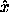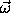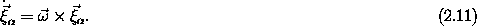## 2.2  Kinematics of Rotation

The motion of a rigid body about a center of rotation, a reference position that is fixed with respect to the body, is characterized at each moment by a rotation axis and a rate of rotation. Let's elaborate.

We can get from any orientation of a body to any other orientation of the body by a rotation of the body. That this is true is called Euler's theorem on rotations about a point.2 We know that rotations have the property that they do not commute: the composition of successive rotations in general depends on the order of operation. Rotating a book about theaxis and then about theaxis puts the book in a different orientation than rotating the book about theaxis and then about theaxis. Nevertheless, Euler's theorem states that however many rotations have been composed to reach a given orientation, the orientation could have been reached with a single rotation. Try it! We take a book, rotate it this way, then that, and then some other way -- then find the rotation that does the job in one step. So a rotation can be specified by an axis of rotation and the angular amount of the rotation.

If the orientation of a body evolves over some interval of time, then the orientation at the beginning and the end of the interval can be connected by a single rotation. In the limit that the duration of the interval goes to zero the rotation axis approaches a unique instantaneous rotation axis. And in this limit the ratio of the angle of rotation and the duration of the interval approaches the instantaneous rate of rotation. We represent this instantaneous rotational motion by the angular velocity vector, which points in the direction of the rotation axis (with the right-hand rule giving the direction of rotation about the axis) and has a magnitude equal to the rate of rotation.

If the angular velocity vector for a body isthen the velocities of the constituent particles are perpendicular to the vectors to the constituent particles and proportional to the rate of rotation of the body and the distance of the constituent particle from the instantaneous rotation axis:Isn't it interesting that we have found a concise way of specifying how the orientation of the body is changing, even though we have not yet described a way to specify the orientation itself?

2 For an elementary geometric proof see Whittaker , p. 2.